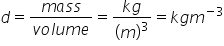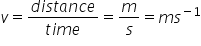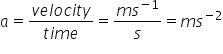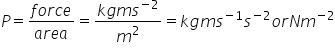# Measurement

Unit of measurements:-

Fundamental units are those which are neither derived from one another nor can be dissolved. Eg. Units of mass, there are 7 basic units of measurement.

Systems of units:-

• CGS system:- in which  units of length, mass and time, in cm, gram & seconds.
• FPS:- in which fundamental meter, units in foot, kilogram, pound & second.
• MKS:- meter, kilogram & second.
• SI unit:-

In SI system (System international d units)

SI units for seven basic quantities:-

 Name of quantity Symbol Name of SI unit Symbol for SI unit Length L Meter M Mass M Kilogram Kg Time t Second S Temperature T Kelvin K Electric current I Ampere A Luminous intensity Iv Candela Cd Quantity of matter N Mole Mol

Derived units:- these are obtained from basic units:-

There are:-

1. Area = length x  breadth = m x m = m2
2. Volume:-  V = length x breadth x height

= m x m x m = m3

iii. Density (d) =iv) Velocity (v)v) Acceleration (a)vi) Force (f)           f = mass x acceleration = kg ms-2                unit of force = Newton

(vii) PressureIts unit is also called as Pascal (Pa)

Symbols of commonly used prefixes in power of ten

 Deci. (d) 10-1 Deca. (da) 101 Conti. (c) 10-1 Hecto. (h) 102 Milli. (m) 10-3 Kilo. (K) 103 Micro. (µ)10-6 Mega. (M) 106 Mono. (η) 10-9 Giga (G) 109 Pico (P) 10-12 Tetra (T) 1012 Fento (f) 10-15 Peta (P) 1015 Alto (a) 10-18 Exa (E)  1015 Zepto (z) 10-21 Zeta (Z) 1021 Yocto (y) 10-24 Yotta (Y) 1024

Some other inter conversions:-

1 mile = 1.6 km = 1.6 x 103 m

1 hour = 60 x 60 = 3600 s

1 foot = 12 inches

1 inch = 2.5 x 10-2m = 2.5 cm

1 pound = 0.453 kg = 453 g

1 m3 = 100 x 100 x 100 cm3

1 m3 = 106 cm3

Mass and weight:-

Mass:- mass of a substance is the amount of matter present in it and it is constant.

Weight:- it’s the force exerted by gravity on an object. It may vary from one place to another.

Significant figures (S.F)

The significant figures in any number are all the certain digits with last digit uncertain or doubtful.

Rules for reporting S.F:-

1. All non-zero digits in a number are significant.
2. The zeros between two non-zero digits are always significant.
3. The zeros written to the L.H.S of first non-zero digit in a number are not significant.
4. All the zeros placed on right of decimal point are significant.

Note:- if any number ends in zero without any decimal, then zeros may or may not be significant.

Example:-  in (i) 44.3               S.F 3

(ii) 5.243               S.F 4

(iii) 44.2                S.F 3

6.01 has        3 S.F

6.001 has     4 S.F

0.523 has     3 S.F

0.002 has     1 S.F

0.016 has     2 S.F

11.0 has        3 S.F

15.00 has     4 S.F

16.000 has   5 S.F

The no. 25000 may have 2,3,4 or 5 S.F so to remove this confusion scientific notation is used i.e. N x 104

Where N => a no. with single non-zero digit to the left of decimal point.

N => exponent of 10, it may be +ve, -ve, or zero.

25000 can be written as

2.5 x 104       has         2 S.F

2.50 x 104     has         3 S.F

2.500 x 104   has         2 S.F

2.5000 x 104        has         2 S.F

Related Keywords